Home News About Me Ph. D. Thesis Abstract Table of Contents 1. Introduction 2. Theory  Fundamentals of the Multivariate Data Analysis 3. Theory  Quantification of the Refrigerants R22 and R134a: Part I 4. Experiments, Setups and Data Sets 5. Results  Kinetic Measurements 6. Results  Multivariate Calibrations 6.1. PLS Calibration 6.1.1. Wald-Wolfowitz Runs Test 6.1.2. Durbin-Watson Statistics 6.1.3. Results of Statistical Tests 6.2. Box-Cox Transformation + PLS 6.3. INLR 6.4. QPLS 6.5. CART 6.6. Model Trees 6.7. MARS 6.8. Neural Networks 6.9. PCA-NN 6.10. Neural Networks and Pruning 6.11. Conclusions 7. Results  Genetic Algorithm Framework 8. Results  Growing Neural Network Framework 9. Results  All Data Sets 10. Results  Various Aspects of the Frameworks and Measurements 11. Summary and Outlook 12. References 13. Acknowledgements Publications Research Tutorials Downloads and Links Contact Search Site Map Print this Page## 6.1.   PLS Calibration

PLS1 models were built for the calibration data set of the refrigerants (see section 4.5.1.1) with Martens' Uncertainty Test , for the determination of the insignificant variables and with the recommended determination of the number of principal components (factors) according to: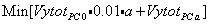(25)

with a as the current dimensionality (principal component number), Vytot as the total residual y-variance validated with a principal components respectively 0 principal components (total y-variance). This means that for each principal component 1% of the total y-variance is added to the variance at principal component number a. This ensures that only principal components are added, which significantly improve the model.

For R22 a model was calibrated with 2 principal components and for R134a a model with 3 principal components. Martens' Uncertainty Test removed no variable for R22 and only the time points 15 s and 67 s for R134a. In figure 28, the x and y loadings are shown for R134a. It is visible that nearly all variables are highly correlated whereby the time points during desorption form a cluster (top right) and the time points during sorption form a second cluster (bottom left). The three dots near the origin of coordinates are the first 3 time points during sorption and the remaining 2 dots are the fist 2 time-points during desorption. The corresponding loadings plot for R22 is analogous with only the location of the two clusters exchanged.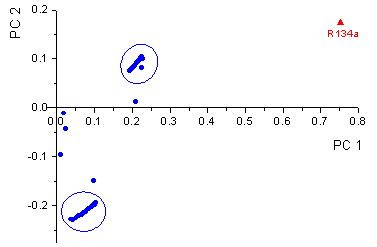figure 28:  Loadings plot of the x and y variables for the calibration of R134a.

The relative root mean square errors of the predictions of the calibration data are very high with 11.89% for R22 and 11.40% for R134a. The prediction of the independent validation set also showed disappointing high relative errors of 10.27% for R22 and 9.94% for R134a. In figure 29 the predictions of the validation data are shown as true-predicted plots. It is visible that the plots show a curvature and consequently the predictions are highly biased. The curved true-predicted plots are a typical sign of nonlinearities in the data, which are not successfully calibrated by a linear model. In figure 30, the T scores are plotted versus the U scores of the model of R134a for the first principal component, which explains 92.5% of the y-variance and 54.2% of the x-variance. The different concentration levels of R134a are visible along the axis of the U scores and the different concentrations of the interfering analyte R22 are visible as scattering along the axis of the T scores. As this type of plot shows the inner relationship of the PLS model (see expression (10)), the nonlinearities, which are visible in figure 30 and which are also visible in the less scattered T scores versus U scores plots for the higher principal components, demonstrate that the linear PLS model cannot deal with a nonlinear relationship present in the data. The corresponding plots for R22 are analogous and will not be discussed here.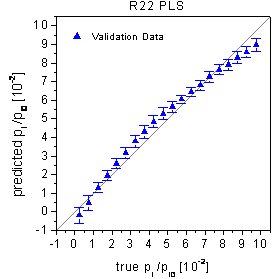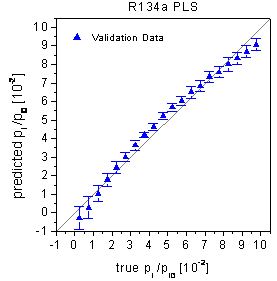figure 29:  True-predicted plots of the PLS calibration for the validation data. The optimal number of principal components was determined by Martens' Uncertainty. The predictions are poor due to systematic biases.figure 30:  T scores versus U scores for the first principal component of the model for the calibration of R134a.

It is known , - that the linear PLS sometimes can be successfully applied to nonlinear problems when minor important higher principal components are included into the model. These principal components may encapsulate not only noise but also significant information about the nonlinear relationship. Thus, the minimum crossvalidation error was used as selection criterion for the optimal number of principal components, as this criterion is less conservative in terms of the number of principal components compared to expression (25). The optimal models contain 5 components for R22 and 6 components for R134a, which were selected using the minima in figure 31. The calibration data were predicted with relative RMSE of 10.47% for R22 and 8.51% for R134a. The predictions of the validation data are visualized in figure 32 with relative RMSE of 8.69% for R22 and 7.63% for R134a. Although the increasing number of principal components improved the errors of prediction, the plots show that the improvement can be put down to less scattered predictions but not to an improved calibration of the nonlinearities. The true-predicted plots can also explain why the predictions of the calibration data are worse than the predictions of the validation data. At both ends of the concentration range, the predictions are most biased. As the concentration range of the calibration data is wider (pi/pio=0-0.1) than the concentration range of the validation data (pi/pio=0.05-0.095) the high bias at the lower and upper concentration limit significantly increases the RMSE of the prediction of the complete calibration data set.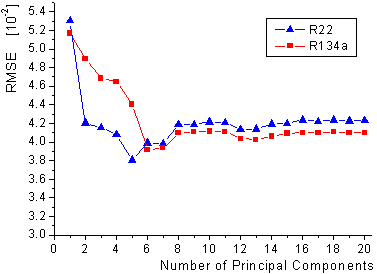figure 31:  Crossvalidated root mean square errors for the first 20 principal components for the calibration of R22 and R134a.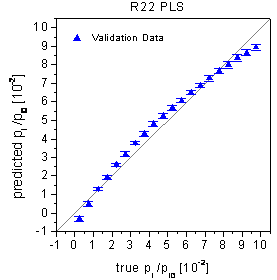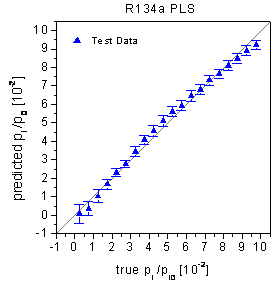figure 32:  True-predicted plots of the PLS for the validation data. The optimal number of principal components was determined by a full crossvalidation of the calibration data. The predictions are still rather poor visible as systematic biases.

In order to find a statistical basis to evaluate the ability of a method to calibrate nonlinearities, statistical methods can be used, which investigate the randomness of residuals of sequential observations. Theses tests like the Durbin-Watson statistics were originally developed for sequential observations equidistant in space or time. Centner et al.  showed that the Durbin-Watson Statistics can be used to test the residuals of purity data without a space or time component while implementing the test into a SIMPLISIMA algorithm for a mixture analysis. In this study, the mean residuals in figure 29 and figure 32 are treated with these statistics by using the increasing concentration levels as "virtual equidistant space component". The Durbin-Watson statistics is used to investigate the correlation of the residuals and additionally the Wald-Wolfowitz Runs test is used to test if the signs of the residuals occur in a random sequence.

 Page 87 © Frank Dieterle, 03.03.2019